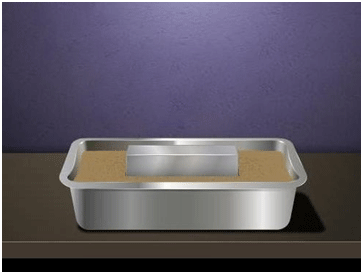Pressure Exerted by a Solid Iron Cuboid on Sand

# Our Objective

To observe and compare the pressure exerted by a solid iron cuboid on sand while resting on its three different faces and to calculate the pressure exerted in the three cases.

# The TheoryTo study and compare the pressure exerted by a solid iron cuboid on sand, we need to find its mass and weight.

## Can you define the Mass of an object?

The mass of an object is a fundamental property of the object; a numerical measure of its inertia; a fundamental measure of the amount of matter in the object. The usual symbol for mass is 'm' and its SI unit is kilogram.

In everyday usage, mass is often referred to as weight, the units of which are often taken to be kilograms. In scientific use, weight is the gravitational force acting on a given body, while mass is an intrinsic property of this body.

On the surface of the Earth, the weight W of an object is related to its mass m by W = m.

## Having defined Mass, what about the Weight of an object?

In science, the weight of an object is the force on the object due to gravity. Its magnitude (a scalar quantity), often denoted by W, is the product of the mass m of the object and the magnitude of the local gravitational acceleration g. Thus, W = mg. Since the weight is a force, its SI unit is Newton.

Simply stated, weight is the force acting vertically downward. The weight of an object is the force with which it is attracted towards the earth, that is:For an object in free fall, when gravity is the only force acting on it, the expression for weight follows Newton's Second Law.

W = F, thus:Here ‘g’ is the Earth's gravitational field strength, equal to about 9.81 m s−2.

An object's weight depends on its environment, while its mass does not. The SI unit of weight is the same as that of force, that is, Newton (N).The force acting on an object perpendicular to the surface is called thrust. The effect of thrust depends on the area on which it acts. Thus:The thrust on unit area is called pressure. Thus:SI unit of pressure is N/m2 or Nm-2 (Newton per square metre).

In honour of scientistBlaise Pascal, the SI unit of pressure is called pascal, denoted by Pa.

# Learning outcomes

1. The depression in sand is greater when the solid iron cuboid is placed on its least surface area.
2. The pressure exerted by the smallest surface area is greater than the other surfaces with larger areas.
3. Thus, the students understood the theories of force, area pressure, depression and their dependence on each other.